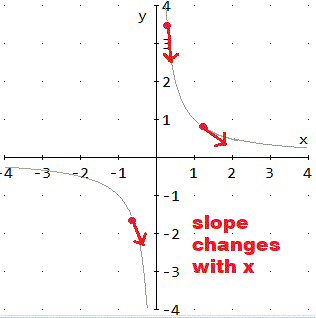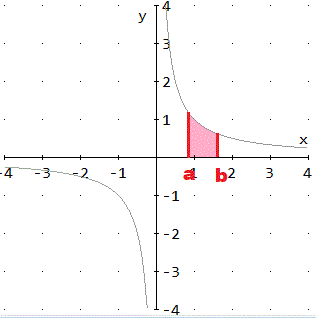## Parent Functions, Their Slope Functions, and Area Functions

 Functions Dislpayed on This Page     The function names in words and the symbols for the function link to their graph, their slope and its graph, and their area function and its graph. the identity function, x the opposite function, -x the reciprocal function, 1/x a constant function, y = c, c = some constant the "twice" function, y = 2x, as in two times the number the squaring function, y = x2 the square root function, y =x, or x1/2 the absolute value function, |x| an exponential or power function, cx where c > 0 and c1,       for example, 23 means 2·2·2 the exponential function, exp(x) or ex a lograthmic functions, logc(x), c > 0 the natual log function, ln(x) or loge(x)

The Identity Function, x
Function, f(x), y: y = x
 x =Slope Function, m, f'(x), dy/dx: m = 1
 x =Area Function, from a to b, isx2/2, from a to b
 a =      b =The Opposite Function, -x
Function, f(x), y: y = -x
 x =Slope Function, m, f'(x), dy/dx: m = - 1
 x =Area Function, from a to b, is- x2/2, from a to b
 a =      b =1. What's the reciprocal of the reciprocal? * 1/(1/x) = x, the identify function*
The Reciprocal Function, 1/x
Function, f(x), y: y = 1/x
 x =Slope Function, m, f'(x), dy/dx: m = -1/(2x2)
 x =Area Function, from a to b, isln|x|, from a to b
 a =      b =1. In the case below, what's the value of c, the constant?*c is 2*
A Constant Function, y = c, c = some constant
Function, f(x), y: y = c, c = some constant
 x =      c=Slope Function, m, f'(x), dy/dx: m = 0
 x =     c=Area Function, from a to b, isc*|b-a|, from a to b
 a =      b =     c =The "Twice" Function, y = 2x, as in two times the number
Function, f(x), y: y = 2x
 x =Slope Function, m, f'(x), dy/dx: m = 2
 x =Area Function, from a to b, isx2, from a to b
 a =      b =The Squaring Function, y = x2
Function, f(x), y: y = x2
 x =Slope Function, m, f'(x), dy/dx: m = 2x
 x =Area Function, from a to b, isx3/3, from a to b
 a =      b =The Square Root Function, y =x, is x1/2
Function, f(x), y: y =x, is x1/2
 x =Slope Function, m, f'(x), dy/dx: m = 1/(2(x)), x > 0
 x =Area Function, from a to b, is2*(x3)/3, from a to b
 a =      b =The Absolute Value Function, |x|
Function, f(x), y: y = |x|
 x =x = If x is 0, the slope is 0.Area Function, from a to b, isx2/2, from a to b
 a =      b =1. In this case, what is the value of c, the base, used in the graphs?* c is 2*
An Exponential or Power Function, cx where c > 0 and c1, for example, 23 means 2·2·2
Function, f(x), y: y = cx
 x =     c =Slope Function, m, f'(x), dy/dx: m = cxln(c)
 x =     c = c>0Area Function, from a to b, iscx/ln(c), from a to b
 x =     c =  , c>0     a =     b =1. How does the function in this table compair to the function in the last table?
*Above the base is c. Below the base is e.*
2. What is the value of ln(e)?*It is 1.*
The Exponential Function, exp(x) or ex
Function, f(x), y: y = exp(x) or ex
 x =Slope Function, m, f'(x), dy/dx: m = ex
 x =Area Function, from a to b, isex, from a to b
 a =     b =Visit the Interactive Sketch Pad Material On The function ex, Its Derivative, & Its Integral Dere2x -- Derivative of the exponential e2xSums -- The Antiderivative of ex

3. How does the slope function in this table compair to the slope function in the last table?
*Above m = cxln(c). Below m = exln(e) which is ex.*
4. How does the area function in this table compair to the area function in the last table?
*Above area = cx/ln(c). Below area = ex/ln(e) which is ex.*
5. What may be said about the function, the slope function, and the area function in the above table?
*They are each ex.*

1. What is a log, logarithm? *A log is an exponent.*
2. The symbols "log2(8)" is read "the exponent to which 2 must be raised in order to get 8."
What is the value of log2(8)?
*3, because 23 = 8*
3. What is c, the base of this log function? *c = 2*
A Lograthmic Functions, logc(x)
Function, f(x), y: y = logc(x)
 x =       c =Slope Function, m, f'(x), dy/dx: m = 1/(xln(c))
 x =       c =Area Function, from a to b, is[xln(x) - x ]/ln(c), from a to b
 c =      a =      b =1. What is the value of log10(10)?*It is 1.*
2. What is the value of ln(e)?*It is 1.*
The Natual Log Function, ln(x) or loge(x)
Function, f(x), y: y = ln(x)
 x =Slope Function, m, f'(x), dy/dx: m = 1/x
 x =Area Function, from a to b, is[ xln(x) - x ], from a to b
 a =      b =In Closing ...     Hope you had some fun.     For more about "Exponential or Power Functions," "A Bit about e, the base of the Natural Logs," or "The Exponential Function," visit this page in the Function and Relation Library.